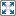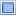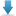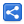# Python - Lists

[Last Updated: Mar 9, 2022]

Python list is ordered collection which can have duplicates.

### Declaring list

```even_numbers = [2, 4, 6]
print(even_numbers)```
`[2, 4, 6]`

### Declaring list of mixed types

```items = [2, "three", 2.3]
print(items)```
`[2, 'three', 2.3]`

### Using type() on list

```even_numbers = [2, 4, 6]
print(type(even_numbers))```
`<class 'list'>`

### Accessing list elements

Lists can be accessed via zero-based indexing:

```even_numbers = [2, 4, 6]
print(even_numbers)
print(even_numbers)
print(even_numbers)```
`246`

### Accessing list from right side

Negative indexing can be used to access the list from right side:

```even_numbers = [2, 4, 6]
print(even_numbers[-1])
print(even_numbers[-2])
print(even_numbers[-3])```
`642`

### Finding index of an element

To get zero-based index we can use `list.index(x[, start[, end]])`

```alpha_list = ['a', 'b', 'c', 'd', 'e']
i = alpha_list.index('c')
print(i)```
`2`

### Finding index in sub-list

```alpha_list = ['a', 'b', 'a', 'c', 'a']
i = alpha_list.index('a', 2) # from index 2
print(i)
i = alpha_list.index('a', 3, 5) # from index 3 to index 5
print(i)```
`24`

### ValueError

ValueError is raised if element does not exist when we use index() method:

```alpha_list = ['a', 'b', 'c', 'd', 'e']
i = alpha_list.index('z')
print(i)```
`Traceback (most recent call last):     i = alpha_list.index('z')ValueError: 'z' is not in list`

### Getting length of a list

The built-in function `len()` can be used to find the length of a list:

```even_numbers = [2, 4, 6]
length = len(even_numbers)
print(length)
```
`3`

### Replacing list element

To change value of an element at specified index we can just assign a new value to that specific index container:

```even_numbers = [2, 4, 6]
even_numbers = 10
even_numbers = 12
even_numbers = 14
print(even_numbers)```
`[10, 12, 14]`

### IndexError

If we try to access a list out side of it's index range:

```even_numbers = [2, 4, 6]
print(even_numbers)```
`Traceback (most recent call last):     print(even_numbers)IndexError: list index out of range`

if we try to add a new element by using index outside of it's index range:

```even_numbers = [2, 4, 6]
even_numbers=8
print(even_numbers)```
`Traceback (most recent call last):     even_numbers=8IndexError: list assignment index out of range`

### Appending new elements

We can use append() method of list object to add new element at the end:

```even_numbers = [2, 4, 6]
even_numbers.append(8)
print(even_numbers)```
`[2, 4, 6, 8]`

### Inserting new elements

We can use `list.insert(i, x)` method of list object to insert new element at the specified index:

```even_numbers = [2, 4, 6]
even_numbers.insert(0, 10)
print(even_numbers)```
`[10, 2, 4, 6]`

### Inserting from right side

```even_numbers = [2, 4, 6]
even_numbers.insert(-1, 10)
print(even_numbers)```
`[2, 4, 10, 6]`

### Appending elements from other list

The method `list.extend(iterable)` can be used to add another list to this list:

```even_numbers = [2, 4, 6]
even_numbers2 = [12, 14, 16]
even_numbers.extend(even_numbers2)
print(even_numbers)```
`[2, 4, 6, 12, 14, 16]`

### Appending elements using concatenation

The concatenation of two list with + returns a new instance of the list:

```even_numbers = [2, 4, 6]
even_numbers2 = [12, 14, 16]
new_numbers = even_numbers + even_numbers2
print(new_numbers)```
`[2, 4, 6, 12, 14, 16]`

### Removing elements by index

The method `list.pop([i])` removes the item at the specified index and returns it. If we don't specify any index, element will be removed at the end

```even_numbers = [2, 4, 6]
item = even_numbers.pop(0)
print(even_numbers)
print(item)

# without any index
odd_numbers = [1, 3, 5]
item = odd_numbers.pop()
print(odd_numbers)
print(item)```
`[4, 6]2[1, 3]5`

### Removing elements by index from right

We can do that by using negative index with `pop()` method:

```even_numbers = [2, 4, 6]
even_numbers.pop(-1)
print(even_numbers)```
`[2, 4]`

### Removing elements by item values

The method `list.remove(x)` can be used to remove a specified item:

```even_numbers = [2, 4, 6]
even_numbers.remove(4)
print(even_numbers)

# in case we have multiple items with same values
alpha_list = ['a', 'b', 'c', 'b']
alpha_list.remove('b')
print(alpha_list)
alpha_list.remove('b')
print(alpha_list)```
`[2, 6]['a', 'c', 'b']['a', 'c']`

### Removing elements by del keyword

The del keyword can also be used to removed a specified item:

```alpha_list = ['a', 'b', 'c', 'e']

# removing at index 0
del alpha_list
print(alpha_list)

# removing at index from right
del alpha_list[-1]
print(alpha_list)```
`['b', 'c', 'e']['b', 'c']`

### Removing whole list

```alpha_list = ['a', 'b', 'c', 'd', 'e']
#removing complete list (just like other objects via del)
del alpha_list
print(alpha_list)```
`Traceback (most recent call last):     print(alpha_list)NameError: name 'alpha_list' is not defined`

### Clearing all elements

`list.clear()` can be used to remove all elements from the list:

```alpha_list = ['a', 'b', 'c', 'd', 'e']
alpha_list.clear()
print(alpha_list)```
`[]`

### Looping list

```even_numbers = [2, 4, 6]
for x in even_numbers:
print(x)
```
`246`

### looping by index

```even_numbers = [2, 4, 6]
length = len(even_numbers)
for x in range(length):
print(even_numbers[x])```
`246`

### Checking if an element exists in a list

```alpha_list = ['a', 'b', 'c', 'd', 'e']
result = 'c' in alpha_list
print(result)```
`True`

### Finding frequency of an element

The occurrence or count or frequency can be found by using count() method:

```alpha_list = ['a', 'b', 'a', 'd', 'a']
i = alpha_list.count('a')
print(i)
# if element does not exist
i = alpha_list.count('z')
print(i)```
`30`

### Finding substrings

[a:b] can be used to find sub-subsequence (substring) of a list:

```alpha_list = ['a', 'b', 'c', 'd', 'e']
print(alpha_list[1:3])
print(alpha_list[:2])
print(alpha_list[2:])

# using del
del alpha_list[2:4]
print(alpha_list)
```
`['b', 'c']['a', 'b']['c', 'd', 'e']['a', 'b', 'e']`

### Sorting

The method `list.sort(key=None, reverse=False)` can be used to sort:

```num = [9, 1, 5, 3, 8]
num.sort()
print(num)```
`[1, 3, 5, 8, 9]`

### Sorting in reverse order

Use `list.sort(reverse=True)` to sort in reversed order:

```num = [9, 1, 5, 3, 8]
num.sort(reverse=True)
print(num)```
`[9, 8, 5, 3, 1]`

### Custom sorting by key

Following example shows how to use key to customize sorting:

```# Custom sorting by key
alpha = ['a', 'B', 'b', 'A', 'c']
alpha.sort(key=str.lower)
print(alpha)

# without key=str.lower
alpha2 = ['a', 'B', 'b', 'A', 'c']
alpha2.sort()
print(alpha2)

# custom key function
def even(i):
return i % 2 == 0

num = [2, 3, 5, 6]
num.sort(key=even)
print(num)
```
`['a', 'A', 'B', 'b', 'c']['A', 'B', 'a', 'b', 'c'][3, 5, 2, 6]`

### Reversing order

We can use list method `list.reverse()` to reverse the order:

```num = [9, 1, 5, 3, 8]
num.reverse()
print(num)```
`[8, 3, 5, 1, 9]`

### Copying list

The method `list.copy()` returns the shallow copy of this list:

```alpha = ['a', 'b', 'c']
alpha2 =alpha.copy()
print(alpha2)

#checking ids
print(id(alpha))
print(id(alpha2))```
`['a', 'b', 'c']19295439152641929544170112`

## Example Project

Dependencies and Technologies Used:

• Python 3.10.2
 Python - List ExamplesSelect AllDownload• python-list-examples
• scripts
• 01_declaring_list.py

 Share